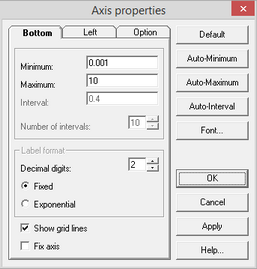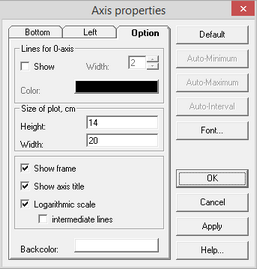﻿ ANSDIMAT+ > Diagnostic plot > Plot view > Axis properties

Axis properties

Navigation:  ANSDIMAT+ > Diagnostic plot > Plot view >

Axis properties

The dialog box "Axis properties" enables modifications of plot axes attributes."Axis properties" dialog box

Explanatory table

 "Bottom" tab Modifications for the vertical (y) axis setup "Left" tab Modifications for the vertical (x) axis setup "Minimum" text box Changes the axis minimum value "Maximum" text box Changes the axis maximum value "Interval" text box Length of the interval between two marks on the axis "Number of intervals" text box Number of marks on the axis "Label format" frame "Decimal digits" text box Number of decimals "Fixed" option Fixed format of axis labels (e.g. 0.45) "Exponential" option Scientific format of axis labels (e.g. 4.5E-0.1) "Show grid lines" option Displays or hides grid lines "Fix axis" option When redraw a plot (for example, the same plot with other observation wells), the values of the coordinate axis (minimum, maximum, and step) do not change "Option" tab "Show lines for 0-axis" option Highlight the vertical and horizontal lines crossing at the origin point "Width" text box Width of the lines "Color" button Color of the lines "Size of plot" frame Dimensions of the plot in centimeters "Height" text box "Width" text box "Show frame" option Draws a plot frame "Show axis title" option Displays on the plot the title of the coordinate axes "Show logarithmic scale" option For plots plotted in the logarithmic coordinates of time, drawdown (recovery) or distance (s - log t, s - log r, log s - log t, log s - log r), the values of the coordinate axis contain the actual value of the parameter, and not its logarithm "Intermediate lines" option Shows intermediate grid lines on a logarithmic plot "Backcolor" button Selects the background color "Default" button Returns all parameters to default values "Auto-Minimum" button Automatic selection of the minimum value on each axis "Auto-Maximum" button Automatic selection of the maximum value on each axis "Auto-Interval" button Automatic selection of the number and length of intervals between 2 marks on each axis "Font" button Font selection